# High School Physics : Understanding Springs

## Example Questions

### Example Question #1 : Harmonic Motion

A spring has a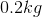mass attached to one, which oscillates with a period of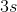. What is the frequency?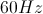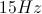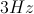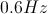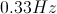Explanation:

The mass has no bearing on the relationship between frequency and period. This relationship is given by the equation: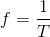Given the period, the frequency will be equal to its reciprocal.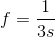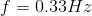### Example Question #1 : Harmonic Motion

A spring with a spring constant of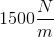is compressed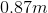. How much potential energy has been generated?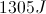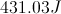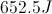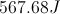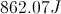Explanation:

The formula for the potential energy in a spring is: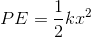Use the given spring constant and displacement to solve for the stored energy.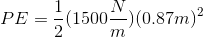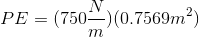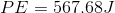### Example Question #3 : Harmonic Motion

A mass is placed at the end of a spring. It has a starting velocity of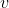and is allowed to oscillate freely. If the mass has a starting velocity of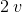, what would the period be?

The period would beas long.

The period would be half as long.

The period would be twice as long.

The period would betimes as long.

The period would remain the same.

The period would remain the same.

Explanation:

When it comes to the period of a spring, the velocity of the object has no effect.

The equation is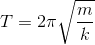.

### Example Question #4 : Harmonic Motion

A spring is attached to a mass, oscillating freely in simple harmonic motion. What change can be made to increase the period of the oscillation?

Increase the mass at the end of the spring

Use a spring with a greater spring constant

Lengthen the spring

Take the spring to a planet with a lower acceleration due to gravity

Decrease the mass at the end of the spring

Increase the mass at the end of the spring

Explanation:

The equation for the period of a spring in simple harmonic motion is:In this formula,is the mass andis the spring constant. The only two things we can adjust that can change the period, then, are the mass and spring constant. The length of the spring and the acceleration due to gravity are irrelevant.

If we increase the mass we get a larger numerator, which in turns will give us a larger period. If we decrease the mass we get a smaller numerator, which would give us a smaller period. If we use a higher spring constant we get a larger denominator, which also gives us a smaller period.

### Example Question #5 : Harmonic Motion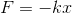The force,, acts downwards

The spring constant,, is always negative

The force,, acts in the opposite direction of the displacement,The force,, acts in a negative direction

The force,, acts in the opposite direction of the displacement,The equation given is Hooke's law, which is used to determine spring force based on the spring constant,, and the displacement of the spring,.Negative and downward are arbitrary, and spring constants are always positive. If a spring is stretched or compressed in a given direction, the force of the spring will always act opposite the direction of the displacement in order to return the spring to the resting equilibrium position.